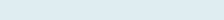# Separate Date and TIME from a Timestamp

After reading this article you will able to change the normal date to Excel date when time is also given in the same cell or substitute date to dd- mm-yyyy format.

## Splitting when Timestamp is Text

Query: When you have a column of dates with time. Our task is to extract date or time separately then use the below formula. When the date is in Text format or not recognized by Excel.

Formula to implement:

=DATE(MID(B4,SEARCH(“/”,B4)+4,4),LEFT(B4,SEARCH(“/”,B4)-1),MID(B4,SEARCH(“/”,B4)+1,2))

Function applicable

Date: The Excel DATE function returns a date value using year, month and date sequentially.

Mid: The Excel MID function extracts a specified number of characters from the middle of a given text string.

Search: The Excel SEARCH function finds the position of a specified character or sub-string within a text string. The return value depends upon the starting character (start_num) of the text string (within_text).

Left: The Excel LEFT function displays the number of characters specified in the argument of text starting from the left.

Formula explanation (step by step)

You can also evaluate the formula, just click the F9 function key in Excel.

Let’s break the formula into multiple small steps to understand its application.

First Formula:

SEARCH(“/”,B4)

Syntax: =SEARCH(find_text,within_text,[start_num])

Step 1:Result: 2

It will first search “/” in cell B4 so as it is the second number it returns 2.

Second Formula:

MID(B4,SEARCH(“/”,B4)+4

Syntax: MID (text, start_num, num_chars)

Step 2: MID(“5/27/2020 4:33:46 PM”,6,4)

Result: 2020

As in the first step, we get 2 so 2+4 =6 so, It will return 4 characters starting at 6th positions of a given cell (B4).

Third Formula:

LEFT(B4,SEARCH(“/”,B4)-1)

Syntax: LEFT (text, [num_chars])

Step 3: SEARCH(“/”,B4)-1)

Result: 1

First, it returns 2 as “/” as it comes at 2nd position then subtracts 1.

Third Formula:

LEFT(B4,SEARCH(“/”,B4)-1)

Syntax: LEFT (text, [num_chars])

Step 4: LEFT(“5/27/2020 4:33:46 PM”,1)

Result: 5

Returns 1st position starting the left side of cell B4 – 5

Step 5: SEARCH(“/”,B4)+1,2)

Result: 3

Once again, we will find “/” at position 2 after adding 1 it shows 3.

Step 6: MID(B4,SEARCH(“/”,B4)+3,2))

Result: 27

After indicating 3rd position 2 characters of cell B4 return – 27

Fourth Formula:

DATE(MID(B4,SEARCH(“/”,B4)+4,4),LEFT(B4,SEARCH(“/”,B4)-1),MID(B4,SEARCH(“/”,B4)+1,2))

Syntax: DATE(year, month, day)

Step 7: =DATE(“2020″,”5″,”27”)

Result: 27-05-2020 (users format)

As we already extract date, month, and year so it simply returns a given date after solving the formula.